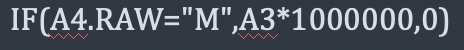# Calculation

Hi There,

I was try to calculate A4 with a number and result place in A6. it works if the field is “Free Text” but if I have it a “Numeric”, the calculation does not work.

Below is the formula.
IF(A4==“M”,A3*1000000,“Wrong”)

Regards
Raymond

Hi,

When the field type is “Free text”, will the formula return calculation if it’s true? Numeric field can only be filled in numbers, can not return string like “Wrong”.

Hi,
Thanks for the note, I also try to change to formula to:
IF(A4==“M”,A3*1000000,0) and the results are the same, No output when the field name is set as numeric.

but if the field name is set as ‘Free Text’ then it does the calculation.

but my problem is that it does not come with the comma on the numbers which I want the results be:

Regards
Raymond

Hi,

You can try